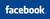Follow AllBankingSolutions  @#### Recurring Deposit Calculator  - India

RD Calculator

Recurring Deposit scheme is offered by almost all banks (RD schemes of SBI, PNB, ICICI Bank, HDFC Bank, IDBI Bank, Bank of India, Bank of Baroda, Corporation Bank  ) in one form or the other.  Recurring Deposit is very popular among the salaried class, specially who can afford to save only few hindered or say few thousand rupees per month.   This scheme is a boon for people who do not have a large amount of savings and thus can not use the Fixed Deposit scheme of the banks.  Under this scheme, the customer deposits a minimum amount (normally fixed) every month, and bank pays the interest at the pre-determined rates (which is usually the  same as applicable to fixed deposits ).  At the end of the period i.e. on maturity date, the customer is paid the maturity value i.e. principle deposited and the interest payable.

Recurring Deposit Calculator - RD Calculator
 Monthly Instalment Amount Rs. Annual Interest Rate % Period in Month(s) - (Must be divisible by 3)
 Maturity Amount (Approx) Rs. Interest Amount (included in Maturity Value) Rs.

Recurring Deposit Interest Calculator

The above is a RD Interest Calculator which calculates the maturity value (i.e. principal amount + interest earned ) of the deposits made under recurring deposit schemes of banks in India.   The above calculator does not account for TDS as different banks will be using different periods for deduction of the TDS (see below that TDS has become applicable now on RD accounts).

TDS On Interest Earned on Recurring Deposits / TDS Rules for RD Interest :

Until May 2015, banks were not required to deduct TDS on the interest earned on RD accounts.    However, wef 1st June, 2015, Tax Deduction at Source (TDS) is applicable on interest paid / accrued on RD accounts.    In case the deposit holder does not want the Tax to be deducted at source, they are required to  submit Form 15G / 15H (other than company, firm or co-operative society)/ Exemption Certificate under Section 197 (in case of all holders)/ or any other Tax Exemption Certificate with the Bank. Such form or tax exemption certificate has to be submitted every financial year for each recurring deposit held with the Bank.  Thus, now the TDS rules for RD are similar to FD rules for TDS.

How Recurring Deposit Interest is calculated ? / Formula for RD Interest calculations :

Formula used by Banks in India / Recurring Deposit Calculations / RD Calculations / Recurring Deposit Calculation Formula / RD Formula used by Indian Banks : The following formula is used by Banks in India for calculation of maturity value of the Recurring Deposit wherein the compounding is done on a quarterly basis:

Art You Planning Your Holidays with your Family in India or Abroad.    You can Simply  Fill Form Given  and You are likely to be  called by upto four Tour Operators from  whom you can check the rates and other details.  Compare them and if you like finally book the Travel Agent Which you Like.

 Maturity Value of the Recurring Deposits  - based on  Quarterly Compounding) M =R [ (1+i)n – 1]          --------------------              1- (1+i) -1/3  M = Maturity value R = Monthly installment n = Number of quarters i = Rate of interest/400

In EXCEL ( .xls file ) the Future Value (FV) is used for this purpose.  However, it has been noticed that it does not exactly match with the values as per the bank maturity value charts of banks in India.

Click Here to know :  Highest Rate of Interest on Saving Fund Accounts

You May Be Interested in Reading  Our Three other popular articles relating to Fixed Deposits:

(a)